# ProblemsA 0.2 kg baseball is travelling at 40 m/s. After being hit by a bat, the ball's velocity is 50 m/s in the opposite direction. Find a) the impulse and b) the average force exerted by the bat if the ball and bat are in contact for 0.002 s.

Solution:

a)
Choose the ball's initial direction of motion as the positive direction.
 I = |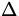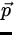| = 0.2(- 50) - 0.2(40) = - 18  kg m/s (13)
b)= I/t = - 18/.002 = - 9000  N (14)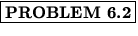A 1000 kg car travelling 22 m/s (about 50 mi/hr) hits a concrete bridge support and comes to a stop in .5 s. a) Find the average force acting on the car and b) if the bridge support had been cushioned so that the stopping time was increased to 3 s, what would have been the average force?

Solution:

a)=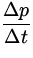= m= 1000- 4.4 x 104  N (15)
b)= 1000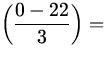- 7.3 x 103  N (16)A 1.0 kg object travelling at 1.0 m/s collides head on with a 2.0 kg object initially at rest. Find the velocity of each object after impact if the collision is perfectly elastic.

Solution:
Given m1 = 1  kg , m2 = 2  kg , v1i = 1  m/s and v2i = 0  m/s we need to find v1f and v2f . We use the conservation of momentum to write:

 m1v1i = m1v1f + m2v2f (1)(1) = (1)v1f + (2)v2f.
Conservation of kinetic energy gives us:m1v1i2 =m1v1f2 + m2v2f2 (1)(12) = (1)v1f2 + (2)v2f2. (17)
We must solve two equations for two unknowns. The first equation gives v2f =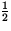-v1f . Substituting into the second equation gives,
 1 = v1f2 + 2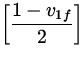2 = v1f2 + 2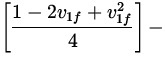1 = 2v1f2 + 1 - 2v1f + v1f2 - 2 = 3v1f2 - 2v1f - 1 = (3v1f + 1)(v1f - 1). (18)
The solutions are v1f = - 1/3  m/s and v1f = 1  m/s . The second solution corresponds to the situation where the two objects miss each other, and the first continues on with it's speed unchanged. The first solution is the one we want. Substituting back into the first equation gives,
 v2f =(1 - v1f) =(1 +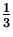) =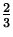m/s. (19)Suppose the collision in the previous problem was completely inelastic. Find a) the velocity of the objects after impact and b) the fraction of the kinetic energy lost during the collision.

Solution:

a)
The conservation of momentum gives,
 m1v1i = (m1 + m2)vf vf =m/s. (20)
b)
Then KEi =1(12) =J and KEf =(1 + 2)(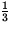2) =J . The percentage of KE that is lost is
 %KElost =100% =x 100% = 66%. (21)In the ballistic pendulum experiment, a bullet of mass .06 kg is fired horizontally into a wooden block of mass .2 kg. The wooden block is suspended from the ceiling by a long string as shown in the diagram. The collision is perfectly inelastic and after impact the bullet and the block swing together until the block is .12 m above it's initial position. Find a) the velocity of the bullet and block just after impact and b) the velocity of the bullet just before impact. See Figure 6.3.

Solution: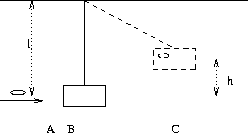Note: In Figure 6.3, point A is just before the bullet strikes the block, point B is just after the bullet strikes the block, and point C is when the bullet and block reach their maximum height.

We want to find vB , the velocity of the bullet and block combination just after impact, and vA , the velocity of the bullet just before impact.

a)
Energy is conserved between points B and C:
 PEC = KEB (m1 + m2)gh =(m1 + m2)vB2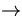vB ==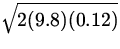= 1.53  m/s. (22)
b)
Momentum is conserved between A and B:
 m1vA + 0 = (m1 + m2)vBvA =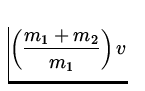B =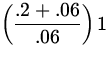.53 = 6.6  m/s. (23)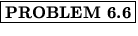Two discs of equal mass are involved in a perfectly elastic glancing collision. The second disc is initially at rest and is struck by the first disc moving to the right at 5.0 m/s. After the collision, the first disc moves in a direction that makes an angle of 37 o with its initial direction, and the second disc moves perpendicularly to the first. Find the speed of each disc after the collision.

Solution: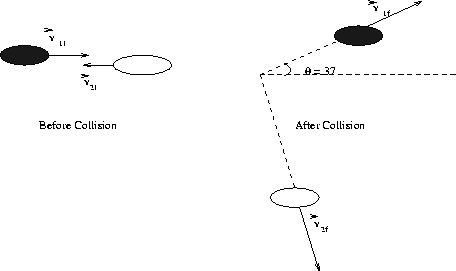We apply the conservation of momentum in the x and y directions.

 x : mv1i = mv1fcos 37 o + mv2fcos 53 o y : 0 = mv1fsin 37 o - mv2fsin 53 o
The y-equation gives v1f = 1.33v2f . Substituting into the x -equation gives,
 5 = (cos 53 o + 1.33cos 37 o )v2fv2f = 3.0  m/s (24)
and,
 v1f = 1.33v2f = 4.0  m/s. (25)A car weighing 1500 kg collides with a van weighing 2500 kg at right angles in the center of an intersection. A detective arrives at the scene and finds that the car and van stuck together and skidded 15 m at 53 o as shown.After pulling out his 1301 text and doing some calculations, he charges the owner of the car with speeding (in a 60 km/hr zone), as well as running through a stop sign. How fast was the car going before going through the collision? Take the coefficient of kinetic friction between the tires and the road to be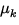= 0.8 .

Solution:

a)
From the length of the skid mark, he can calculate the speed of the car and the van immediately after the collision. First, find the acceleration of the pair of vehicles due to the force of friction.
 Ffr = - (mc + mv)aa = -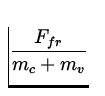= -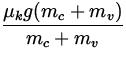= -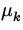g = - 7.84 m/s 2 (26)
Then, using v = 0 (at the end of the skid), and d = 15  m , he can obtain the speed immediately after the collision from,
 v 2 = v02 + 2adv0 =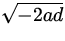=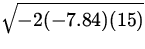= 15.33  m/s. (27)
b)
He can use the conservation of momentum to find the initial velocities. The x -component gives,
 mcvci = (mv + mc)vfcos 53 ovci =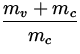vfcos 53 o = 14.76  m/s = 53.2  km/hr (28)
and the y -component gives,
 mvvvi = (mv + mc)vfsin 53 ovvi =vfsin 53 o = 32.7  m/s = 117.6  km/hr > 60  km/hr. (29)